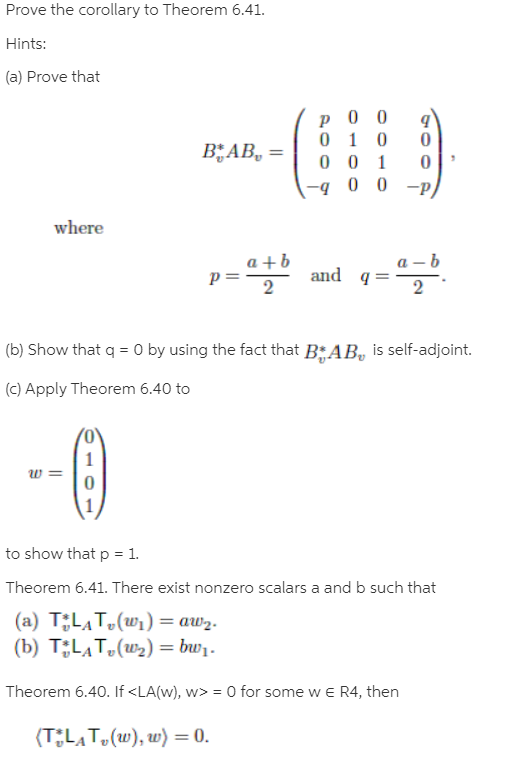# Prove the corollary to Theorem 6.41.Hints:(a) Prove thatBAB, =-q 0 0 -p)wherea +ba – bq =and2(b) Show that q = 0 by using the fact that BAB, is self-adjoint.(c) Apply Theorem 6.40 toto show that p = 1.Theorem 6.41. There exist nonzero scalars a and b such that(a) T;LĄT,(wi) = aw2.(b) T;LAT,(w2) = bw1.Theorem 6.40. If = 0 for some w e R4, then(TLAT,(w), w) = 0.2.

Question
1 viewshelp_outlineImage TranscriptioncloseProve the corollary to Theorem 6.41. Hints: (a) Prove that BAB, = -q 0 0 -p) where a +b a – b q = and 2 (b) Show that q = 0 by using the fact that BAB, is self-adjoint. (c) Apply Theorem 6.40 to to show that p = 1. Theorem 6.41. There exist nonzero scalars a and b such that (a) T;LĄT,(wi) = aw2. (b) T;LAT,(w2) = bw1. Theorem 6.40. If = 0 for some w e R4, then (TLAT,(w), w) = 0. 2. fullscreen

### This question hasn't been answered yet.